# Machine Learning of Bayesian Networks Using Constraint Programming

• Slides: 20Machine Learning of Bayesian Networks Using Constraint Programming Peter van Beek and Hella-Franziska Hoffmann University of Waterloo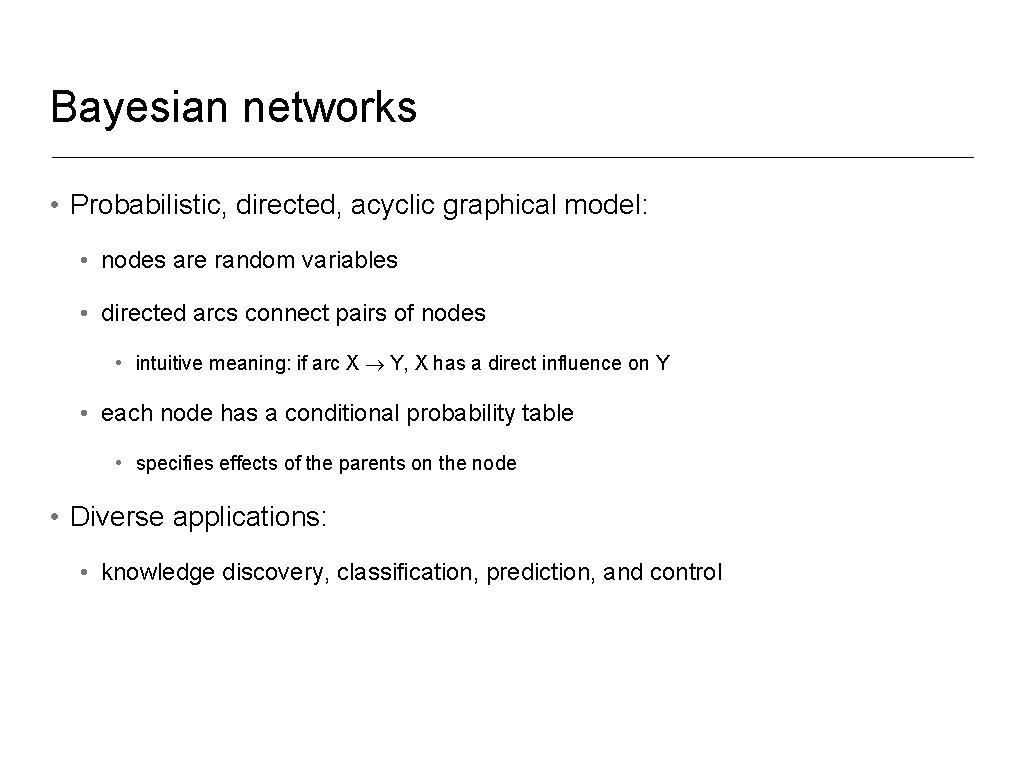Bayesian networks • Probabilistic, directed, acyclic graphical model: • nodes are random variables • directed arcs connect pairs of nodes • intuitive meaning: if arc X Y, X has a direct influence on Y • each node has a conditional probability table • specifies effects of the parents on the node • Diverse applications: • knowledge discovery, classification, prediction, and controlExample: Medical diagnosis of diabetes Heredity Gender Exercise Age Overweight Pregnancies Diabetes Patient information & root causes Medical difficulties & diseases BMI Glucose conc. Serum test Fatigue Diastolic BP Diagnostic tests & symptomsStructure learning from data: score-and-search approach 1. Data: Gender Exercise Age Diastolic BP … Diabetes male yes middle-aged high … yes female yes elderly normal … no … … … 2. Scoring function (BIC/MDL, BDeu) gives possible parent sets: Age Exercise Age Gender 17. 5 20. 2 19. 3 … 3. Combinatorial optimization problem: • find a directed acyclic graph (DAG) over the random variables that minimizes the total score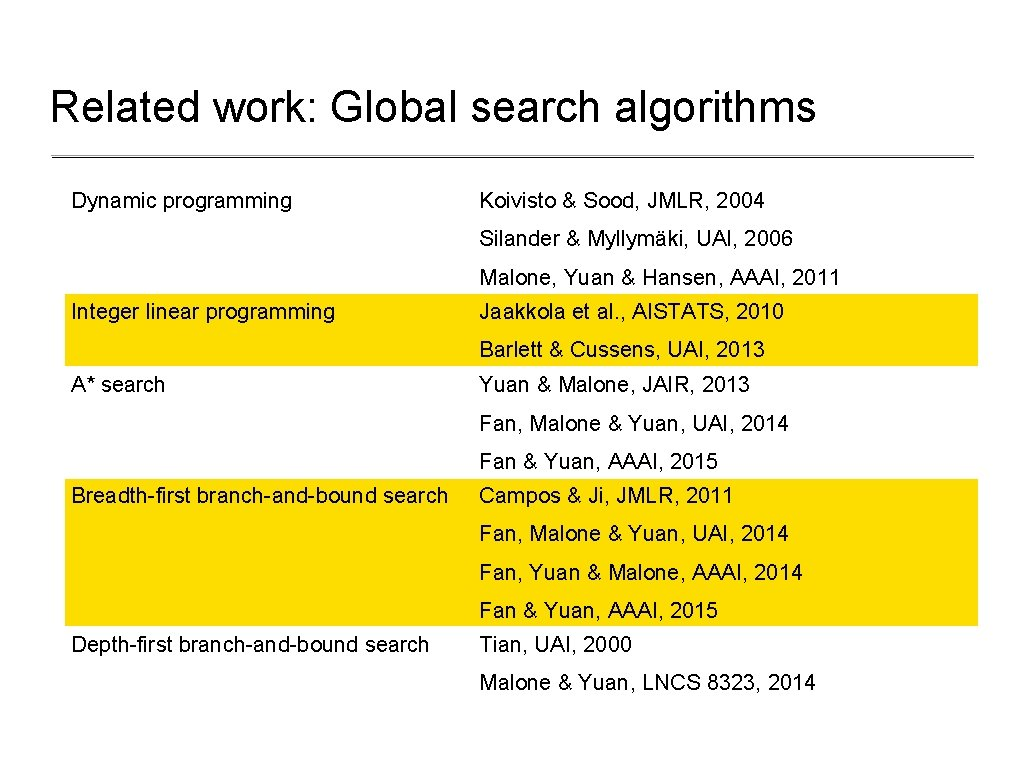Related work: Global search algorithms Dynamic programming Koivisto & Sood, JMLR, 2004 Silander & Myllymäki, UAI, 2006 Malone, Yuan & Hansen, AAAI, 2011 Integer linear programming Jaakkola et al. , AISTATS, 2010 Barlett & Cussens, UAI, 2013 A* search Yuan & Malone, JAIR, 2013 Fan, Malone & Yuan, UAI, 2014 Fan & Yuan, AAAI, 2015 Breadth-first branch-and-bound search Campos & Ji, JMLR, 2011 Fan, Malone & Yuan, UAI, 2014 Fan, Yuan & Malone, AAAI, 2014 Fan & Yuan, AAAI, 2015 Depth-first branch-and-bound search Tian, UAI, 2000 Malone & Yuan, LNCS 8323, 2014Related work: Global search algorithms Dynamic programming Koivisto & Sood, JMLR, 2004 Silander & Myllymäki, UAI, 2006 Malone, Yuan & Hansen, AAAI, 2011 Integer linear programming Jaakkola et al. , AISTATS, 2010 Barlett & Cussens, UAI, 2013 A* search Yuan & Malone, JAIR, 2013 Fan, Malone & Yuan, UAI, 2014 Fan & Yuan, AAAI, 2015 Breadth-first branch-and-bound search Campos & Ji, JMLR, 2011 Fan, Malone & Yuan, UAI, 2014 Fan, Yuan & Malone, AAAI, 2014 Fan & Yuan, AAAI, 2015 Depth-first branch-and-bound search Tian, UAI, 2000 Malone & Yuan, LNCS 8323, 2014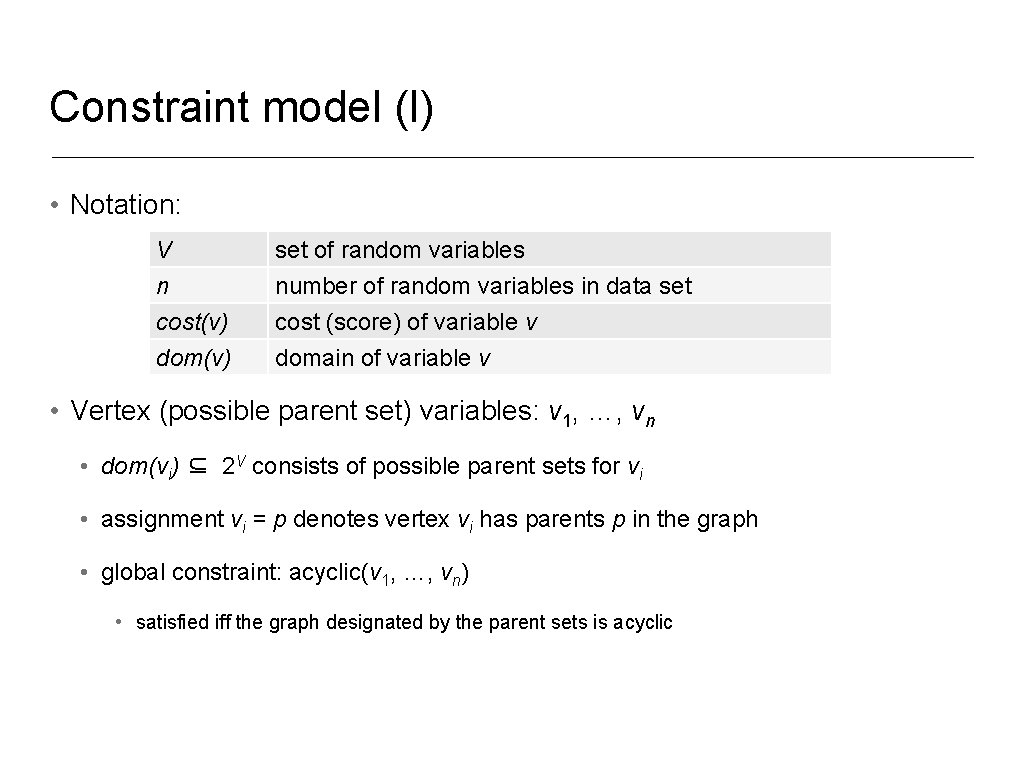Constraint model (I) • Notation: V n cost(v) dom(v) set of random variables number of random variables in data set cost (score) of variable v domain of variable v • Vertex (possible parent set) variables: v 1, …, vn • dom(vi) ⊆ 2 V consists of possible parent sets for vi • assignment vi = p denotes vertex vi has parents p in the graph • global constraint: acyclic(v 1, …, vn) • satisfied iff the graph designated by the parent sets is acyclic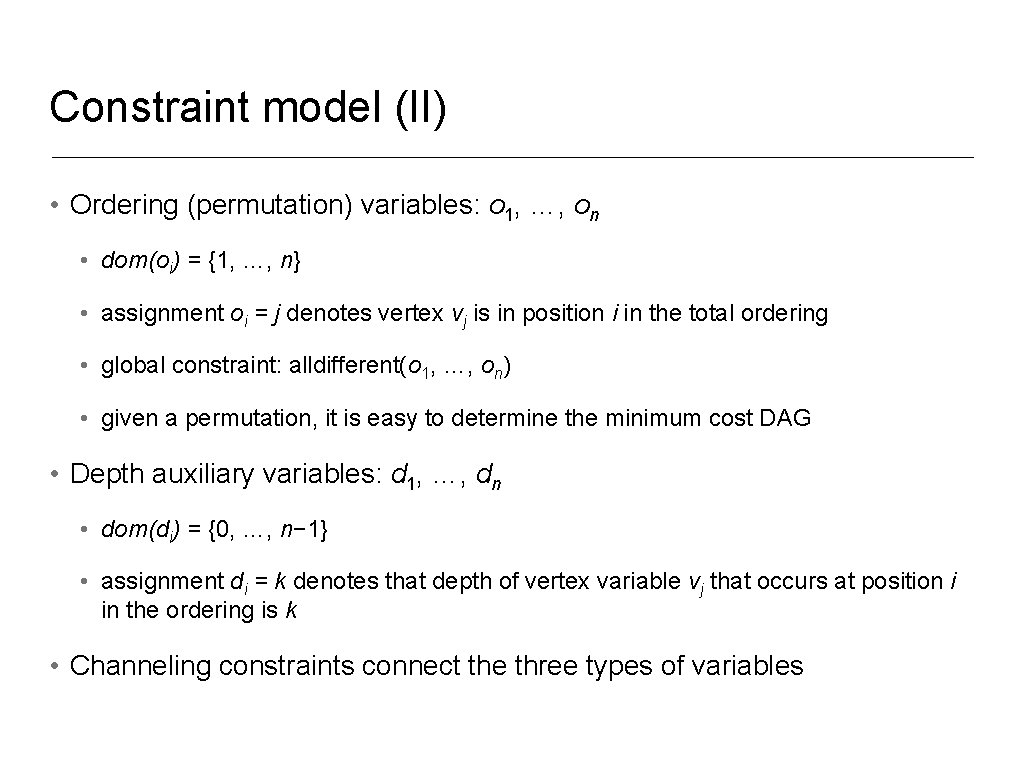Constraint model (II) • Ordering (permutation) variables: o 1, …, on • dom(oi) = {1, …, n} • assignment oi = j denotes vertex vj is in position i in the total ordering • global constraint: alldifferent(o 1, …, on) • given a permutation, it is easy to determine the minimum cost DAG • Depth auxiliary variables: d 1, …, dn • dom(di) = {0, …, n− 1} • assignment di = k denotes that depth of vertex variable vj that occurs at position i in the ordering is k • Channeling constraints connect the three types of variables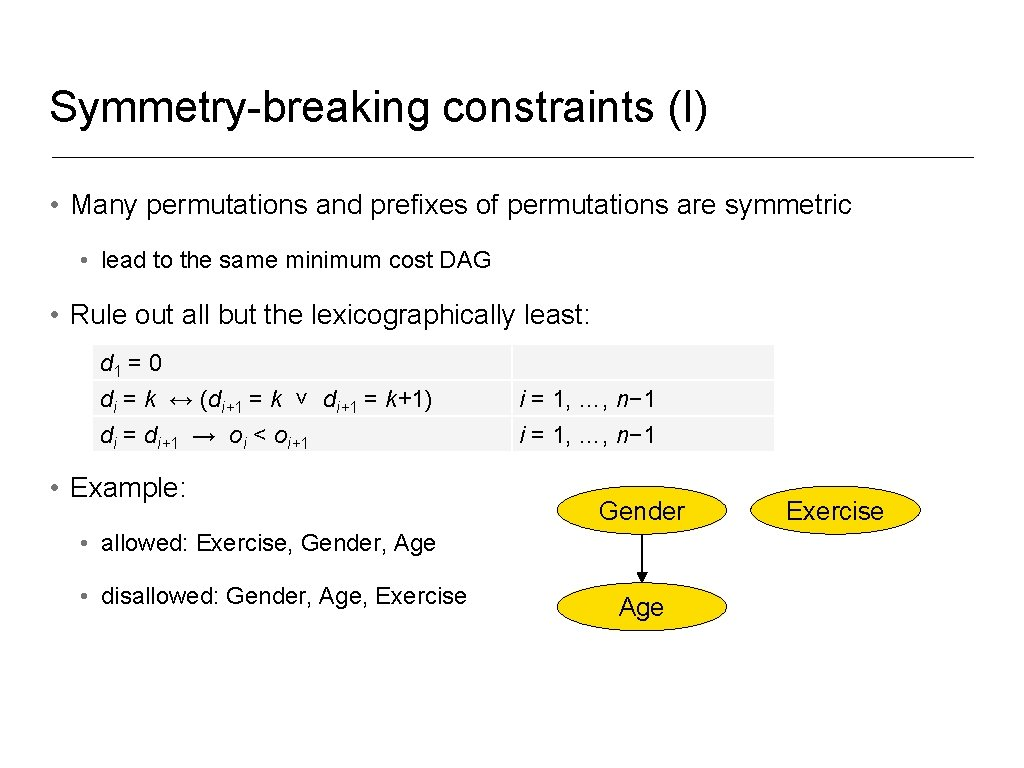Symmetry-breaking constraints (I) • Many permutations and prefixes of permutations are symmetric • lead to the same minimum cost DAG • Rule out all but the lexicographically least: d 1 = 0 di = k ↔ (di+1 = k ˅ di+1 = k+1) di = di+1 → oi < oi+1 • Example: i = 1, …, n− 1 Gender • allowed: Exercise, Gender, Age • disallowed: Gender, Age, Exercise Age Exercise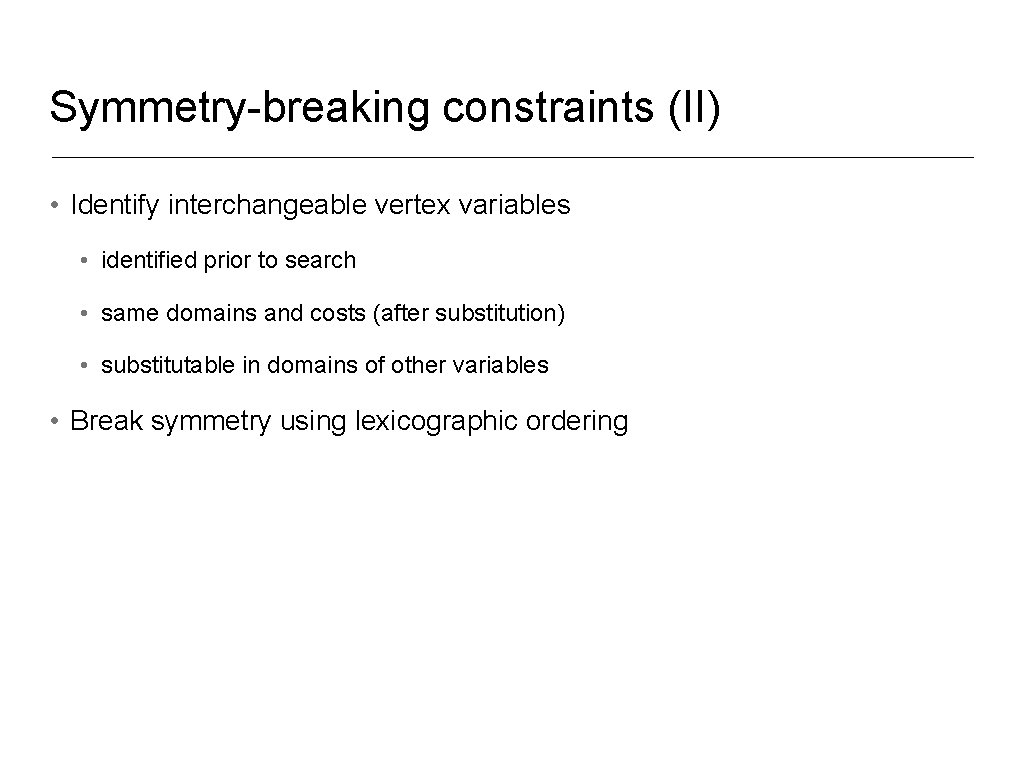Symmetry-breaking constraints (II) • Identify interchangeable vertex variables • identified prior to search • same domains and costs (after substitution) • substitutable in domains of other variables • Break symmetry using lexicographic orderingSymmetry-breaking constraints (III) • I-equivalent networks: • two DAGs are said to be I-equivalent if they encode the same set of conditional independence assumptions • Chickering (1995, 2002) provides a local characterization: • sequence of “covered” edges that can be reversed • Example: Gender Exercise AgeDominance constraints (I) • Consider an instantiation of the ordering prefix o 1, …, oi • A value p ∈ dom(vj) is consistent with the ordering if each element of p occurs in the ordering • want lowest cost p consistent with the ordering • can safely prune away all other p’ ∈ dom(vj) of higher cost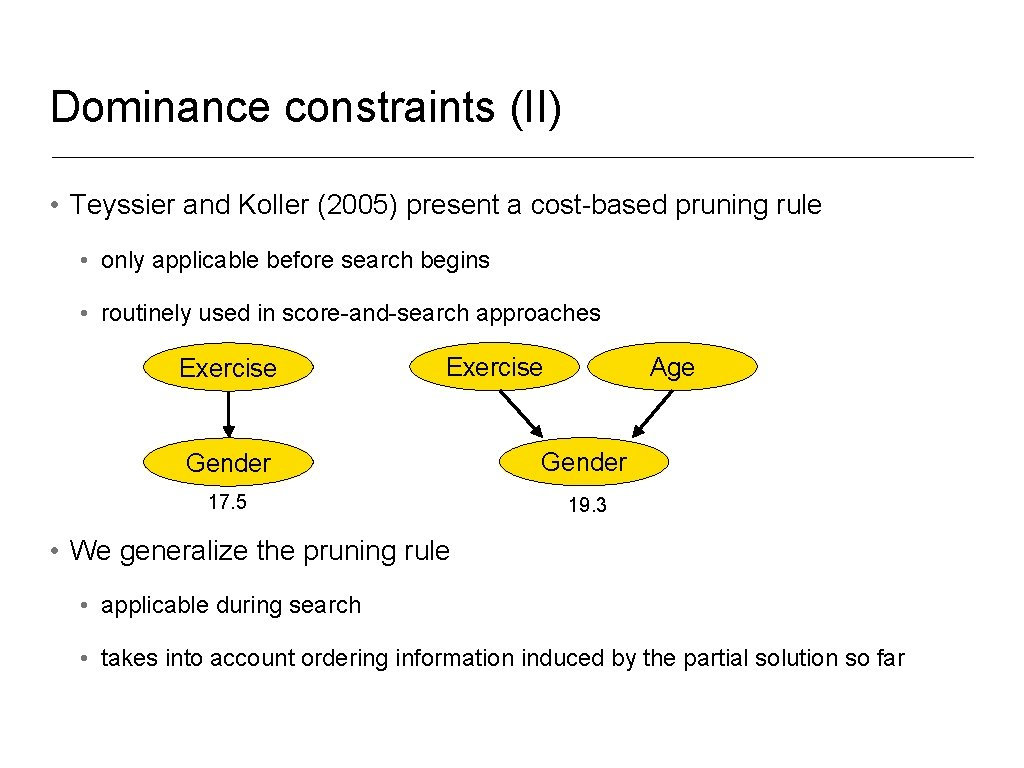Dominance constraints (II) • Teyssier and Koller (2005) present a cost-based pruning rule • only applicable before search begins • routinely used in score-and-search approaches Exercise Age Exercise Gender 17. 5 19. 3 • We generalize the pruning rule • applicable during search • takes into account ordering information induced by the partial solution so far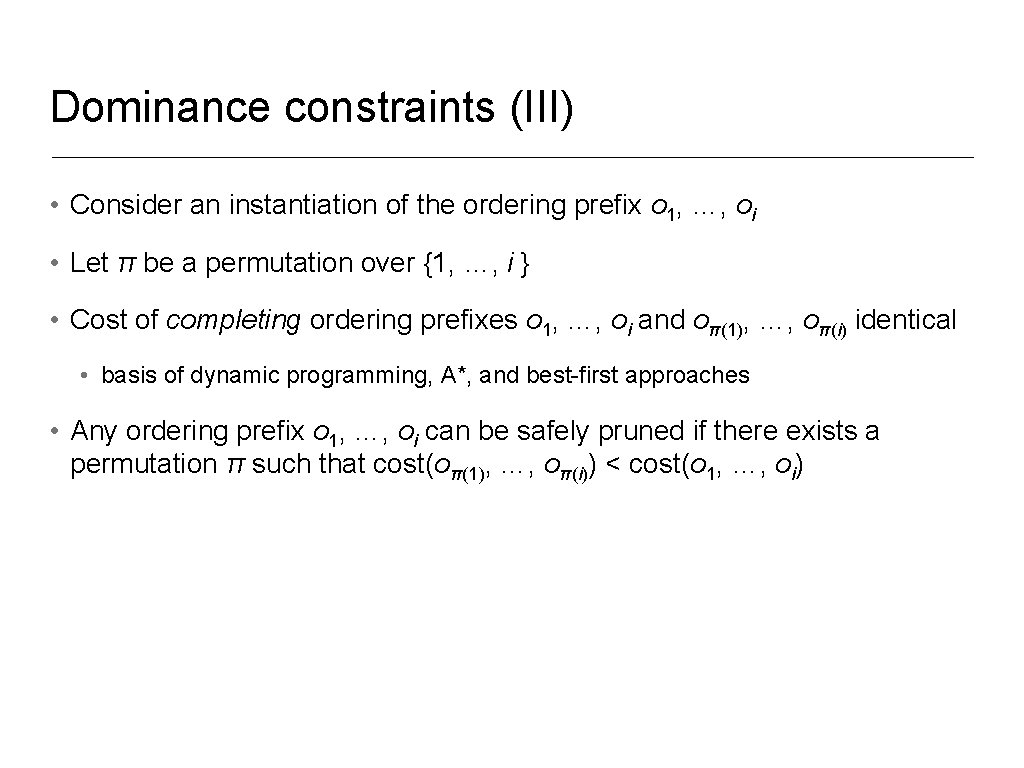Dominance constraints (III) • Consider an instantiation of the ordering prefix o 1, …, oi • Let π be a permutation over {1, …, i } • Cost of completing ordering prefixes o 1, …, oi and oπ(1), …, oπ(i) identical • basis of dynamic programming, A*, and best-first approaches • Any ordering prefix o 1, …, oi can be safely pruned if there exists a permutation π such that cost(oπ(1), …, oπ(i)) < cost(o 1, …, oi)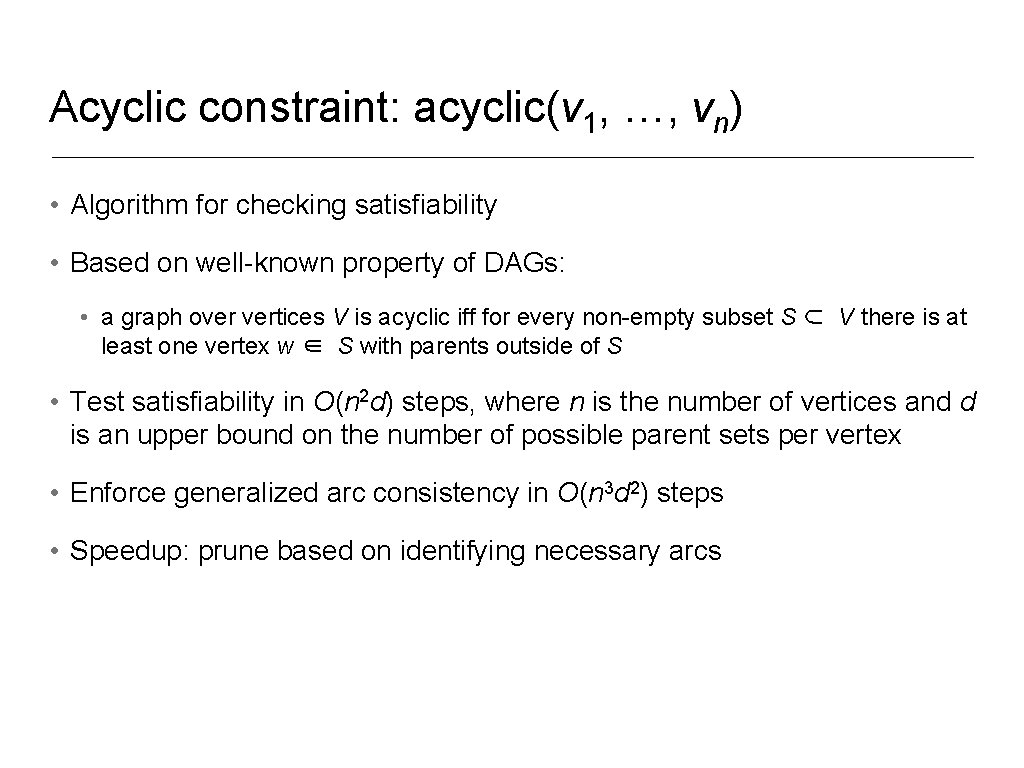Acyclic constraint: acyclic(v 1, …, vn) • Algorithm for checking satisfiability • Based on well-known property of DAGs: • a graph over vertices V is acyclic iff for every non-empty subset S ⊂ V there is at least one vertex w ∈ S with parents outside of S • Test satisfiability in O(n 2 d) steps, where n is the number of vertices and d is an upper bound on the number of possible parent sets per vertex • Enforce generalized arc consistency in O(n 3 d 2) steps • Speedup: prune based on identifying necessary arcs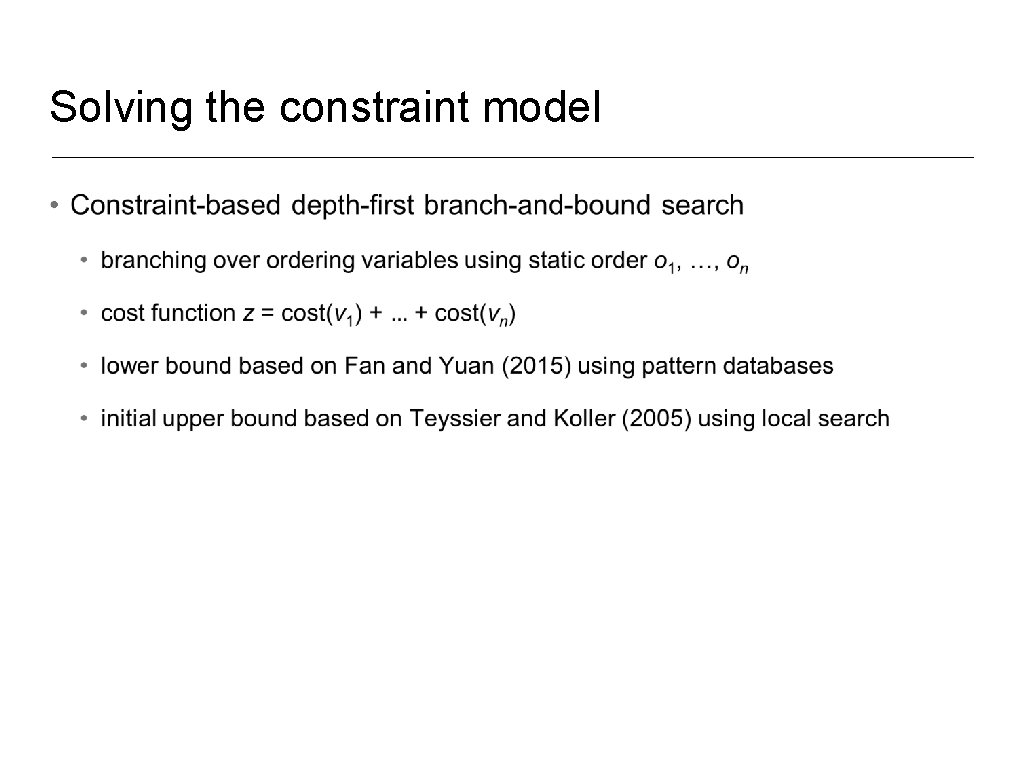Solving the constraint model •Experimental results: BDeu scoring Time (sec. ) to determine minimal cost BN, where n is the number of random variables, N is the number of instances in the data set, and d is the total number of possible parent sets for the random variables. Time limit of 24 hours; memory limit of 16 GB. benchmark n N d GOBNILP A* CPBayes v 1. 4. 1 v 2015 v 1. 0 shuttle 10 58, 000 812 58. 5 0. 0 letter 17 20, 000 18, 841 5, 060. 8 1. 3 1. 4 zoo 17 101 2, 855 177. 7 0. 5 0. 2 vehicle 19 846 3, 121 90. 4 2. 4 0. 7 segment 20 2, 310 6, 491 2, 486. 5 3. 3 1. 3 mushroom 23 8, 124 438, 185 OT 255. 5 561. 8 autos 26 159 25, 238 OT 918. 3 464. 2 insurance 27 1, 000 792 2. 8 583. 9 107. 0 steel 28 1, 941 113, 118 OT 902. 9 21, 547. 0 flag 29 194 1, 324 28. 0 49. 4 39. 9 wdbc 31 569 13, 473 2, 055. 6 OM 11, 031. 6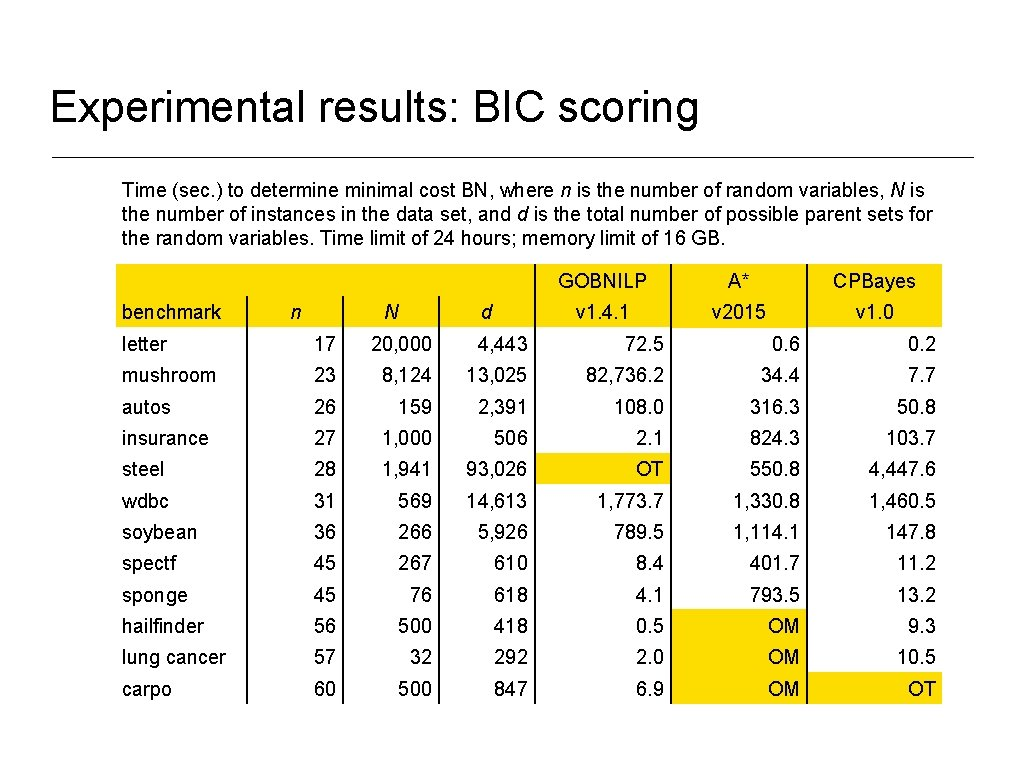Experimental results: BIC scoring Time (sec. ) to determine minimal cost BN, where n is the number of random variables, N is the number of instances in the data set, and d is the total number of possible parent sets for the random variables. Time limit of 24 hours; memory limit of 16 GB. benchmark n N d GOBNILP A* CPBayes v 1. 4. 1 v 2015 v 1. 0 letter 17 20, 000 4, 443 72. 5 0. 6 0. 2 mushroom 23 8, 124 13, 025 82, 736. 2 34. 4 7. 7 autos 26 159 2, 391 108. 0 316. 3 50. 8 insurance 27 1, 000 506 2. 1 824. 3 103. 7 steel 28 1, 941 93, 026 OT 550. 8 4, 447. 6 wdbc 31 569 14, 613 1, 773. 7 1, 330. 8 1, 460. 5 soybean 36 266 5, 926 789. 5 1, 114. 1 147. 8 spectf 45 267 610 8. 4 401. 7 11. 2 sponge 45 76 618 4. 1 793. 5 13. 2 hailfinder 56 500 418 0. 5 OM 9. 3 lung cancer 57 32 292 2. 0 OM 10. 5 carpo 60 500 847 6. 9 OM OT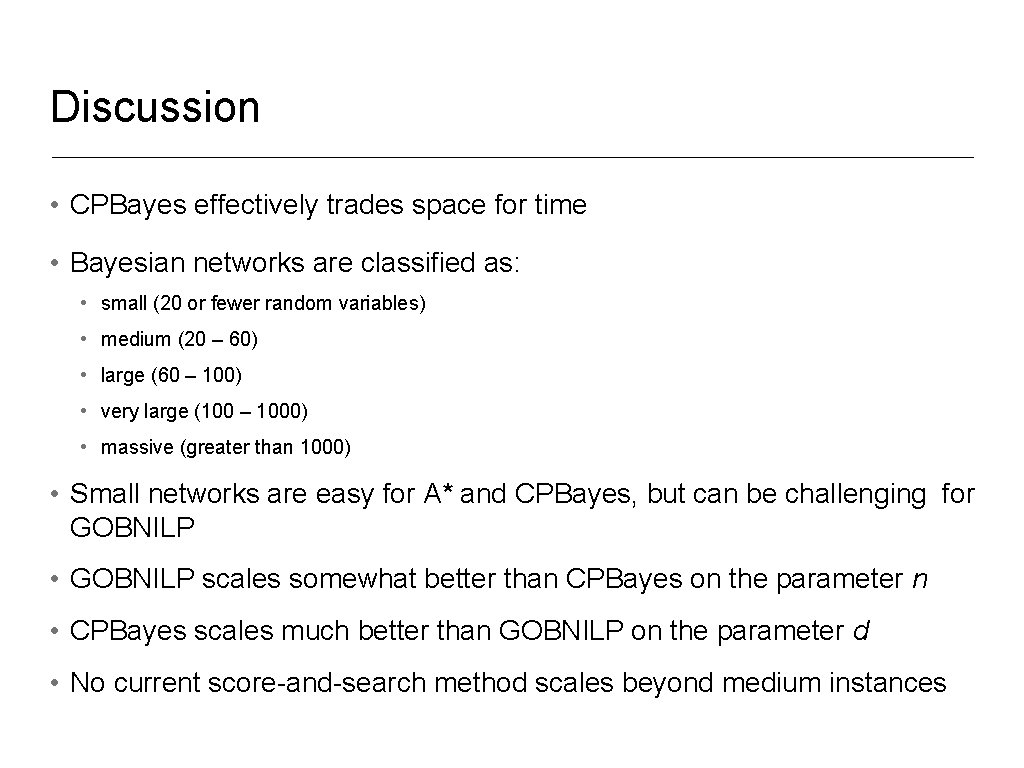Discussion • CPBayes effectively trades space for time • Bayesian networks are classified as: • small (20 or fewer random variables) • medium (20 ‒ 60) • large (60 ‒ 100) • very large (100 ‒ 1000) • massive (greater than 1000) • Small networks are easy for A* and CPBayes, but can be challenging for GOBNILP • GOBNILP scales somewhat better than CPBayes on the parameter n • CPBayes scales much better than GOBNILP on the parameter d • No current score-and-search method scales beyond medium instances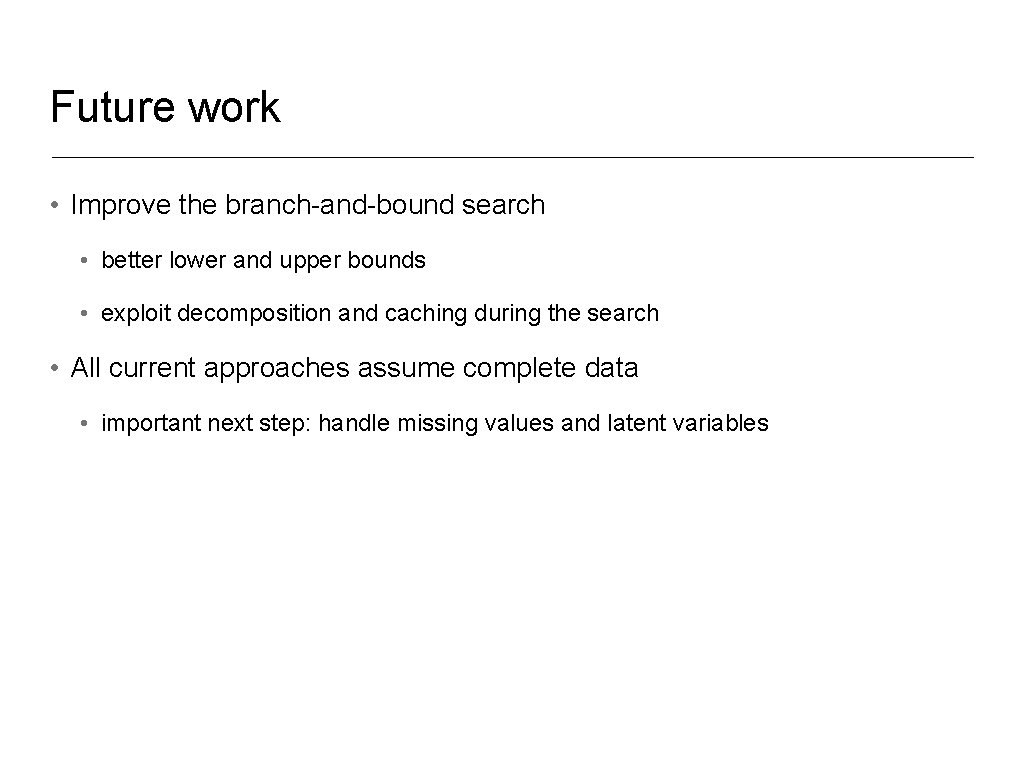Future work • Improve the branch-and-bound search • better lower and upper bounds • exploit decomposition and caching during the search • All current approaches assume complete data • important next step: handle missing values and latent variables